# 十七、更新预测

## “更可能”的二分类器

• 60% 的学生为二年级，其余的 40% 是三年级
• 50% 二年级学生已经声明了他们的专业
• 80% 三年级学生已经声明了他们的专业

## 基于新信息更新预测

``````students.show(3)
``````
Year Major
Second Undeclared
Second Undeclared
Second Undeclared

（省略了 97 行）

``````students.pivot('Major', 'Year')
``````
Year Declared Undeclared
Second 30 30
Third 32 8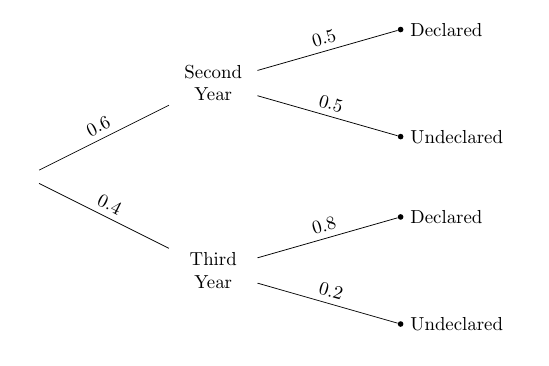``````32/(30+32)
0.5161290322580645
``````

## 树形图

``````students.pivot('Major', 'Year')
``````
Year Declared Undeclared
Second 30 30
Third 32 8

``````(0.4 * 0.8)/(0.6 * 0.5  +  0.4 * 0.8)
0.5161290322580645
``````

### 贝叶斯法则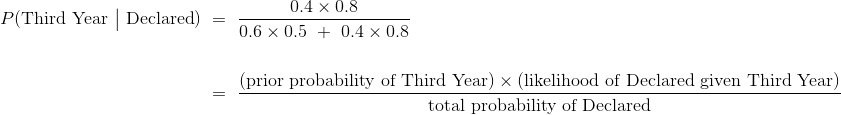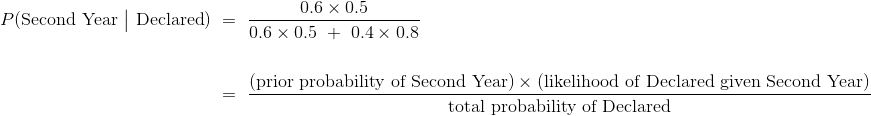``````(0.6 * 0.5)/(0.6 * 0.5  +  0.4 * 0.8)
0.4838709677419354
``````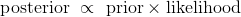## 做出决策

### 罕见疾病的检测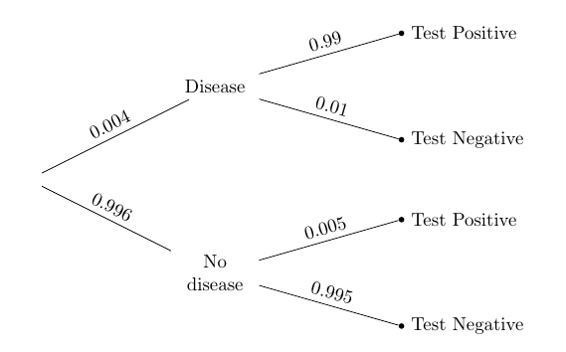``````(0.004 * 0.99)/(0.004 * 0.99  +  0.996*0.005 )
0.44295302013422816
``````

``````population(0.004).pivot('Test Result', 'True Condition')
``````
True Condition Negative Positive
Disease 4 396
No Disease 99102 498

``````396/(396 + 498)
0.4429530201342282
``````### 主观先验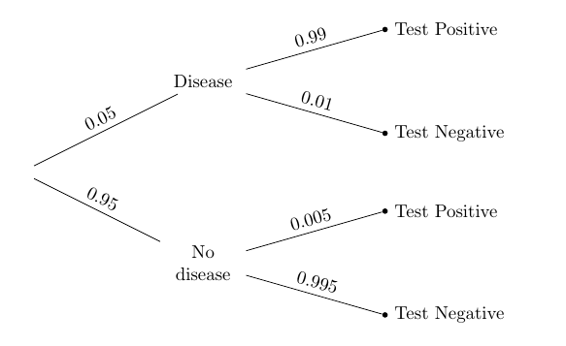``````(0.05 * 0.99)/(0.05 * 0.99  +  0.95 * 0.005)
0.9124423963133641
``````

### 确认结果

``````population(0.05).pivot('Test Result', 'True Condition')
``````
True Condition Negative Positive
Disease 50 4950
No Disease 94525 475

``````4950/(4950 + 475)
0.9124423963133641
``````

``````pop_05 = population(0.05)

sample = pop_05.sample(10000, with_replacement=False)

positive = sample.where('Test Result', are.equal_to('Positive'))
``````

``````positive.where('True Condition', are.equal_to('Disease')).num_rows/positive.num_rows
0.9131205673758865
``````